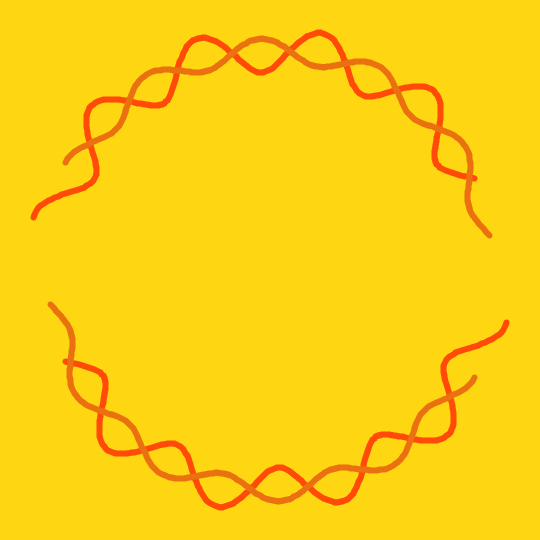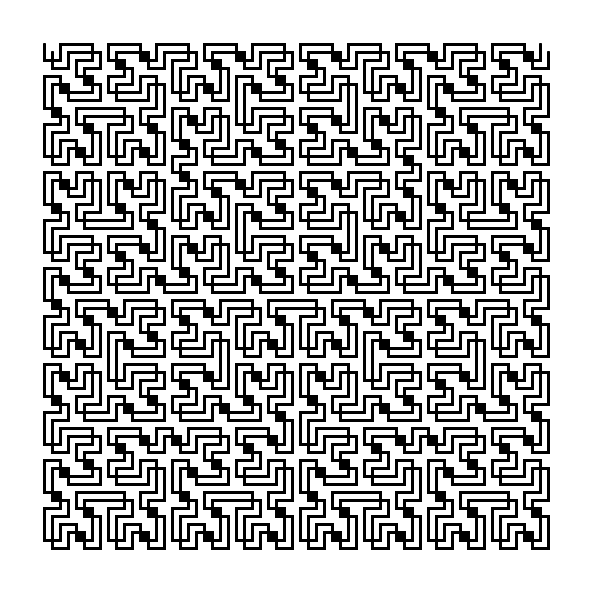Illusion of progress.Illusion of progress.

Flow.Flow.

Flow.Flow.

Two Hilbert curve.Two Hilbert curve.

Two Hilbert curve.Two Hilbert curve.

Finally I had some time to make a new animatio…Finally I had some time to make a new animation. Hopefully more will follow 🙂

Finally I had some time to make a new animatio…Finally I had some time to make a new animation. Hopefully more will follow 🙂

Triangulating a circle. How to do a drawing li…Triangulating a circle.

How to do a drawing like this? Start with a circle and divide it into n equal parts. I choose n=100 for this drawing. There is an easy formula for this, the i-th point is (radius*cos(i*2*PI/n),

radius*sin(i*2*PI/n)). Then for each i connect the i-th point to the point  (radius*cos(PI-2*i*2*PI/n), radius*sin(PI-2*i*2*PI/n)). This might not be one of the original points, but it is on the circle. And that is it 🙂

Why is this mathematically interesting?  This way we get a set of nonparallel  lines such that there are a lot of triple intersections between them.

Triangulating a circle. How to do a drawing li…Triangulating a circle.

How to do a drawing like this? Start with a circle and divide it into n equal parts. I choose n=100 for this drawing. There is an easy formula for this, the i-th point is (radius*cos(i*2*PI/n),

radius*sin(i*2*PI/n)). Then for each i connect the i-th point to the point  (radius*cos(PI-2*i*2*PI/n), radius*sin(PI-2*i*2*PI/n)). This might not be one of the original points, but it is on the circle. And that is it 🙂

Why is this mathematically interesting?  This way we get a set of nonparallel  lines such that there are a lot of triple intersections between them.